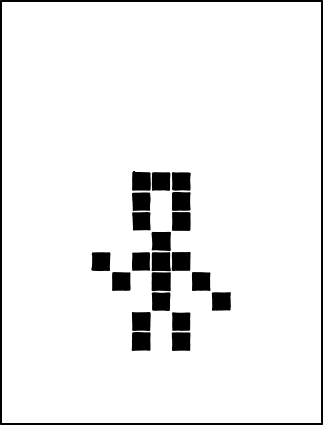more games of life

Posted in Books, Kids, R with tags , , , , on May 5, 2020 by xi'anAnother puzzle in memoriam of John Conway in The Guardian:

Find the ten digit number, abcdefghij. Each of the digits is different, and

• a is divisible by 1
• ab is divisible by 2
• abc is divisible by 3
• abcd is divisible by 4
• abcde is divisible by 5
• abcdef is divisible by 6
• abcdefg is divisible by 7
• abcdefgh is divisible by 8
• abcdefghi is divisible by 9
• abcdefghij is divisible by 10

Which brute force R coding by checking over random permutations of (1,2,…,9) [since j=0] solves within seconds:

while(0<1)
if (prod(!(x<-sum(10^{0:8}*sample(1:9)))%/%10^{7:0}%%2:9))break()

into x=3816547290. And slightly less brute force R coding even faster:

while(0<1){
e=sample(c(2,6,8))#even
o=sample(c(1,3,7,9))#odd
if((!(o+e+o)%%3)&
(!(10*o+e)%%4)&
(!(o+e+o+e+5+4)%%3)&
(!sum(10^{6:0}*c(o,e,o,e,5,4,o))%%7)&
(!(10*o+e)%%8)&
(!(sum(o)+sum(e))%%9)){
print(sum(10^{9:0}*c(o,e,o,e,4,5,o,e,o,0)));break()}}

Le Monde puzzle [#913]

Posted in Books, Kids, Statistics, University life with tags , , , , , , , on June 12, 2015 by xi'anAn arithmetics Le Monde mathematical puzzle:

Find all bi-twin integers, namely positive integers such that adding 2 to any of their dividers returns a prime number.

An easy puzzle, once the R libraries on prime number decomposition can be found!, since it is straightforward to check for solutions. Unfortunately, I could not install the recent numbers package. So I used instead the schoolmath R package. Despite its possible bugs. But it seems to do the job for this problem:

lem=NULL
for (t in 1:1e4)
if (prod(is.prim(prime.factor(t)+2)==1))
lem=c(lem,t)digin=function(n){

which returned all solutions, albeit in a lengthy fashion:

> lem
 1 3 5 9 11 15 17 25 27 29 33 41 45 51 55
 59 71 75 81 85 87 99 101 107 121 123 125 135 137 145
 149 153 165 177 179 187 191 197 205 213 225 227 239 243 255
 261 269 275 281 289 295 297 303 311 319 321 347 355 363 369
 375 405 411 419 425 431 435 447 451 459 461 493 495 505 521
 531 535 537 561 569 573 591 599 605 615 617 625 639 641 649
 659 675 681 685 697 717 725 729 745 765 781 783 807 809 821
 825 827 841 843 857 867 881 885 891 895 909 933 935 955 957
 963 985 1003 1019 1025 1031 ...